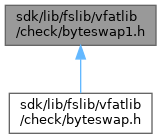ReactOS  0.4.15-dev-3440-g915569a
byteswap1.h File Reference
This graph shows which files directly or indirectly include this file:Go to the source code of this file.

## Macros

#define __bswap_constant_16(x)   ((((x) >> 8) & 0xff) | (((x) & 0xff) << 8))

#define __bswap_constant_32(x)

## Functions

static __inline unsigned short int __bswap_16 (unsigned short int __bsx)

static __inline unsigned int __bswap_32 (unsigned int __bsx)

## ◆ __bswap_constant_16

 #define __bswap_constant_16 ( x ) ((((x) >> 8) & 0xff) | (((x) & 0xff) << 8))

Definition at line 29 of file byteswap1.h.

## ◆ __bswap_constant_32

 #define __bswap_constant_32 ( x )
Value:
((((x) & 0xff000000) >> 24) | (((x) & 0x00ff0000) >> 8) | \
(((x) & 0x0000ff00) << 8) | (((x) & 0x000000ff) << 24))
GLint GLint GLint GLint GLint x
Definition: gl.h:1548

Definition at line 60 of file byteswap1.h.

## ◆ __bswap_16()

 static __inline unsigned short int __bswap_16 ( unsigned short int __bsx )
static

Definition at line 53 of file byteswap1.h.

54 {
55  return __bswap_constant_16 (__bsx);
56 }
#define __bswap_constant_16(x)
Definition: byteswap1.h:29

## ◆ __bswap_32()

 static __inline unsigned int __bswap_32 ( unsigned int __bsx )
static

Definition at line 100 of file byteswap1.h.

101 {
102  return __bswap_constant_32 (__bsx);
103 }
#define __bswap_constant_32(x)
Definition: byteswap1.h:60